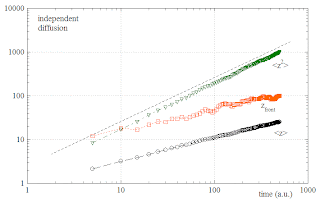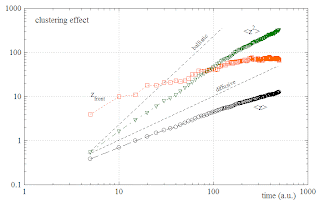## Sunday, August 9, 2009

###  Cell-Invasion : Clustering causes fractional diffusion behaviour ?

One of the most used quantities for the characterization of random walks is the Mean Squared Displacement (MSD) as a function of lagtime, averaged over absolute time. In the case of cell invasion (which might be non-stationary and therefore not suitable for being analyzed with standard MSD), we can define similar quantities of interest as population averages of (powers of) the z-coordinate versus absolute time. For example, we might consider

the Mean Invasion Depth (MID):

\overline{z}(t)=\left\langle z \right\rangle_{pop}=\frac{1}{N}\sum_{n=1}^N z_n(t),

where index n runs over all N agents,

the Mean Squared Invasion Depth (MSID):

\overline{z^2}(t)=\left\langle z^2 \right\rangle_{pop}=\frac{1}{N}\sum_{n=1}^N z^2_n(t),

the Variance (var):

\mbox{var}(t)=\left\langle (z-\overline{z})^2 \right\rangle_{pop}=\frac{1}{N}\sum_{n=1}^N (z_n-\overline{z})^2(t),

and the Invasion Front Position (IFP):

z_{front}(t)=\mbox{max}\left\{ z_n(t) \right\}_{n=1\ldots N}.

Some of these quantities are evaluated in the following for the cell invasion model presented in the last post . We first look at the case of independent random walkers:In the double-logarithmic plot, the MSID is found to grow linearly with time, as would be expected for normal diffusion. The MID, accordingly, grows with the square root of time. The front particle is always ahead of the mean, but is advancing with the same square-root law.

Now we turn to the invasion with clustering:Surprisingly, the MSID grows with time as a fractional, super-diffusive power law ! The MID also grows faster than with the square root of time.

Isn't that counter-intuitive ? How can the occasional slowing down of clustered agents lead, effectively, to a "faster-than-diffusive" invasion dynamics ?

A closer look at the figures reveals that, within the first 100 time units, the normally diffusing agents have invaded - on average - further than the clustering agents, in accordance with common sense. Nevertheless, due to the higher power-law exponent of the clustering agents, there would be a cross-over at some later time. However, the MID and the MSID in the model with clustering seem to show a change of behaviour after about 100 time units. They then seem to return to normal diffusive behaviour, thus avoiding a cross-over.

So the super-diffusion is only a transient effect. It remains surprising, at least for me.